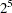## Example 42.2 Factorial Screening

Screening experiments are undertaken to select from among the many possible factors that might affect a response the few that actually do, either simply (main effects) or in conjunction with other factors (interactions). One method of selecting significant factors is forward model selection, in which the model is built by successively adding the most statistically significant effects. Forward selection is an option in the REG procedure, but the REG procedure does not allow you to specify interactions directly (as the GLM procedure does, for example). You can use the GLMMOD procedure to create the screening model for a design and then use the REG procedure on the results to perform the screening.

The following statements create the SAS data set Screening, which contains the results of a screening experiment:

```   title 'PROC GLMMOD and PROC REG for Forward Selection Screening';
data Screening;
input a b c d e y;
datalines;
-1 -1 -1 -1  1  -6.688
-1 -1 -1  1 -1 -10.664
-1 -1  1 -1 -1  -1.459
-1 -1  1  1  1   2.042
-1  1 -1 -1 -1  -8.561
-1  1 -1  1  1  -7.095
-1  1  1 -1  1   0.553
-1  1  1  1 -1  -2.352
1 -1 -1 -1 -1  -4.802
1 -1 -1  1  1   5.705
1 -1  1 -1  1  14.639
1 -1  1  1 -1   2.151
1  1 -1 -1  1   5.884
1  1 -1  1 -1  -3.317
1  1  1 -1 -1   4.048
1  1  1  1  1  15.248
;
run;
```

The data set contains a single dependent variable (y) and five independent factors (a, b, c, d, and e). The design is a half-fraction of the fullfactorial, the precise half-fraction having been chosen to provide uncorrelated estimates of all main effects and two-factor interactions.

The following statements use the GLMMOD procedure to create a design matrix data set containing all the main effects and two-factor interactions for the preceding screening design.

```ods output DesignPoints = DesignMatrix;
proc glmmod data=Screening;
model y = a|b|c|d|e@2;
run;
```

Notice that the preceding statements use ODS to create the design matrix data set, instead of the OUTDESIGN= option in the PROC GLMMOD statement. The results are equivalent, but the columns of the data set produced by ODS have names that are directly related to the names of their corresponding effects.

Finally, the following statements use the REG procedure to perform forward model selection for the screening design. Two MODEL statements are used, one without the selection options (which produces the regression analysis for the full model) and one with the selection options. Output 42.2.1 and Output 42.2.2 show the results of the PROC REG analysis.

```proc reg data=DesignMatrix;
model y = a--d_e;
model y = a--d_e / selection = forward
details   = summary
slentry   = 0.05;
run;
```

Output 42.2.1 PROC REG Full Model Fit
 PROC GLMMOD and PROC REG for Forward Selection Screening

The REG Procedure
Model: MODEL1
Dependent Variable: y

Analysis of Variance
Source DF Sum of
Squares
Mean
Square
F Value Pr > F
Model 15 861.48436 57.43229 . .
Error 0 0 .
Corrected Total 15 861.48436

Root MSE R-Square . 1.0000 0.33325 . .

Parameter Estimates
Variable Label DF Parameter
Estimate
Standard
Error
t Value Pr > |t|
Intercept Intercept 1 0.33325 . . .
a   1 4.61125 . . .
b   1 0.21775 . . .
a_b a*b 1 0.30350 . . .
c   1 4.02550 . . .
a_c a*c 1 0.05150 . . .
b_c b*c 1 -0.20225 . . .
d   1 -0.11850 . . .
a_d a*d 1 0.12075 . . .
b_d b*d 1 0.18850 . . .
c_d c*d 1 0.03200 . . .
e   1 3.45275 . . .
a_e a*e 1 1.97175 . . .
b_e b*e 1 -0.35625 . . .
c_e c*e 1 0.30900 . . .
d_e d*e 1 0.30750 . . .

Output 42.2.2 PROC REG Screening Results

Summary of Forward Selection
Step Variable
Entered
Label Number
Vars In
Partial
R-Square
Model
R-Square
C(p) F Value Pr > F
1 a   1 0.3949 0.3949 . 9.14 0.0091
2 c   2 0.3010 0.6959 . 12.87 0.0033
3 e   3 0.2214 0.9173 . 32.13 0.0001
4 a_e a*e 4 0.0722 0.9895 . 75.66 <.0001

The full model has 16 parameters (the intercept + 5 main effects + 10 interactions). These are all estimable, but since there are only 16 observations in the design, there are no degrees of freedom left to estimate error; consequently, there is no way to use the full model to test for the statistical significance of effects. However, the forward selection method chooses only four effects for the model: the main effects of factors a, c, and e, and the interaction between a and e. Using this reduced model enables you to estimate the underlying level of noise, although note that the selection method biases this estimate somewhat.